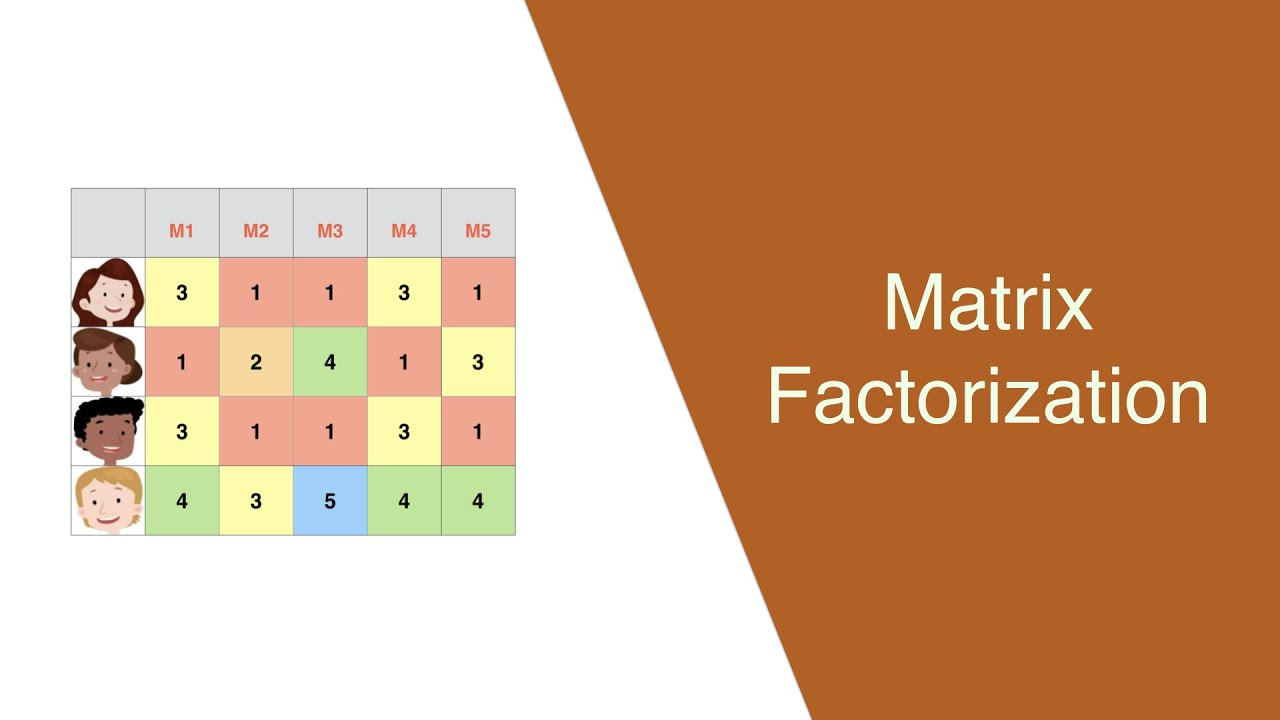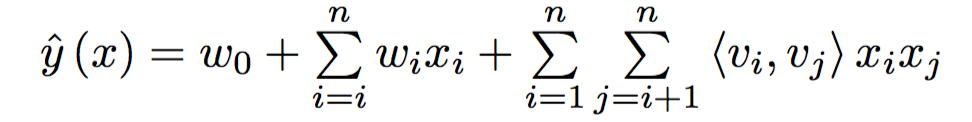# Factorization Machines - 추천 알고리즘

## Intro

Recommender System의 다양한 알고리즘 중 Factorization machines 알고리즘에 대해 살펴보도록 하겠습니다. 이 글은 해당 블로그의 내용을 기반으로 정리하였으며, 저처럼 삽질을 하루 이상 하신 분들에게 도움이 되고자 작성하였습니다. 또한 어려운 수식적인 내용은 가볍게 설명하고 넘어갈테니 직관적인 이해 위주로 받아들여주시면 감사하겠습니다. 혹시 제가 틀린 점이나 보완해야할 부분이 있으시면 꼭 알려주세요.

## Other recommender systems

• Collaborative filtering(협업 필터링)

일반적인 협업 필터링과 Content-based 협업 필터링으로 나눌 수 있다. 전자의 경우 ‘나’와 비슷한 사용자 그룹을 골라내고 그들이 좋은 평점을 준 영화를 추천해주는 방식이다. 후자의 경우 내가 ‘액션’영화를 좋아한다면 내가 아직 보지 못한 액션영화들을 추천해주는 방식이다. 즉, 사람을 기준으로 할 것인지 컨텐츠를 기준으로 할 것인지에 따라 나뉜다고 할 수 있다.

• Matrix Factorization보통 위 그림 중 오른쪽 아래와 같은 테이블을 가지고 있다. 가로 축은 사용자(user), 세로 축은 아이템(item), 그리고 행렬 값에는 그들의 평가(rating)가 기록되어있는 행렬이다. 우리는 4x5 행렬을 각각 4x2, 5x2 행렬로 쪼갤 수 있고 (여기서 2는 임의로 정해지는 차원의 수) 각 행렬을 이루는 벡터의 내적을 통해 rating 값을 얻을 수 있다. 분해된 행렬은 SVD와 같은 수학적인 방식을 이용하거나, gradient descent와 같은 머신 러닝 방식을 이용해 얻을 수 있다.

## Linear regression추천 시스템을 설명할 때 뜬금 없이 회귀라는 말이 왜 나오는 지 필자도 매우 궁금했다. 사실 추천 시스템이라는 것을 인풋(유저, 아이템)에 대한 아웃풋(평가)를 예측한다는 문제라고 생각할 수 있다. 그렇다면 당연히 선형 회귀를 먼저 생각하게 될테고 위 그림과 같이 one-hot encoding 되어있는 features별로 weights를 두어서 문제를 해결하면 된다. 만일 그림에서 user field가 3차원, item feild가 4차원이라면 $w_{1}$부터 $w_{7}$을 구하면 된다.

## Polynomial regression

\begin{align} \hat{y}(\textbf{x}) = w_{0} + \sum_{i=1}^{n} w_{i} x_{i} + \sum_{i=1}^n \sum_{j=i+1}^n x_{i} x_{j} w_{ij} \tag {3} \end{align}

하지만 추천 문제를 선형 회귀적으로만 풀려고 하면 각 feature 사이의 interaction이 무시되는 일이 발생한다. 즉, 2번 유저가 액션영화를 좋아하는 지 등의 관계를 반영하지 못하는 문제가 생기는 것이다. 따라서 단순 선형회귀가 아닌 다중회귀 문제를 풀어야함이 자명하다. 위 식에서 첫 째 항은 global bias, 두 번째 항은 선형 회귀, 세번 째 항이 각 feature 간의 관계를 고려한 부분이다. 각 feature별 관계를 표현할 수 있는 weight $w_{ij}$가 필요하게 된 것이다.

• 여기서 중요한 점은 식의 $x$변수는 feature값(field의 한 차원)이 라는 것이다. 다시 말해 user ‘field’의 벡터가 (1,0,0)이라면 1, 0, 0은 각각 $x_{1}$, $x_{2}$, $x_{3}$이다.

## Factorization machinesFactorization machines 알고리즘은 이름 때문에 Matrix Factorization 방식과 헷갈릴 수 있다. 하지만 둘이 아예 다른 것이라고 생각 하는 것이 마음 편하다. 눈치 빠른 사람들은 벌써 보았겠지만 기존 협업 필터링과 Matrix Factorization 방식에서 나타내는 (user / item / rating)행렬은 가로 축이 user, 세로 축이 item, 값이 rating인 반면 Factorization machines방법은 regression과 본질적으로 같은 방식이기 때문에 행렬 구성에서 가로 축은 index(각 튜플), 세로 축은 field (user, item, …, rating), 값은 각 feature 값을 갖게 된다.

또 재미있는 점은 rating값을 target으로 하는 regression이기 때문에 field를 여러 가지 추가시킬 수 있다는 점이다. 영화로 치면 영화 장르, 배우의 출현 여부 등을 one-hot encoding하여(categorical data의 경우) 넣을 수 있다. 사실 영화와 같은 곳에서 추천은 장르와 같은 metadata가 훨씬 중요한 역할을 한다. 단순 행렬 분해 값을 학습시킨다고 해서 소비자가 좋아하는 장르나 배우를 파악하는 것이 쉽지 않기 때문이다. 따라서 추가적인 feature를 입력할 수 있다는 점에서 매우 유리하다.

하지만 추가적으로 입력하는 feature가 많아질 수록 우리가 계산해야하는 $w_{ij}$는 많아진다. $n(n-1)/2$, 즉 $O(n^2)$의 시간복잡도를 가지게 된다. Factorization machines 알고리즘은 이 문제를 해결함에 있어 행렬이 분해될 수 있음을 이용한다. 즉, NxN의 행렬이 NxK, KxN으로 분해될 수 있으므로 NxK 크기의 행렬을 학습시켜 시간복잡도를 크게 줄일 수 있다.

 Any positive semi-definite matrix W∈Rn×n can be decomposed into VV⊤ (e.g., Cholesky Decomposition). The FM model can express any pairwise interaction matrix W=VV⊤ provided that the k chosen is reasonably large enough. V∈Rk where k≪n is a hyper-parameter that defines the rank of the factorization. 

그렇다면 $w_{ij}$는 어떻게 구할 수 있을까? 바로 NxK 크기의 V 행렬의 i번 째 행과 j번 째 행을 내적하여 얻을 수 있다. 여기서 주의할 점은 N이 각 feature 개수의 총 합이라는 것이다. 즉, user가 10차원, item이 20차원, category가 11차원이라면 N은 10+20+11 = 41이 된다. k는 정보를 충분히 담을 수 있는 수로 정해지고 latent space의 차원이라고 생각할 수 있다. 따라서 우리는 Polynomial regression 문제를 다음과 같이 쓸 수 있다.## Conclusion

우리는 Machine learning으로 rating을 예측하는 것이기 때문에 target과의 MSE error를 loss로 정하고 일반적인 학습 과정을 거쳐 global bias, 선형 회귀 weights, V 행렬의 값들을(parameters) 업데이트 해주면 된다. 추가적으로 feature가 latent vector를 하나만 가지는 문제를 해결한 FFM(Field aware Factorization Machine)방식도 있다고 하니 시간이 나면 찾아봐야겠다.

Categories:

Updated: